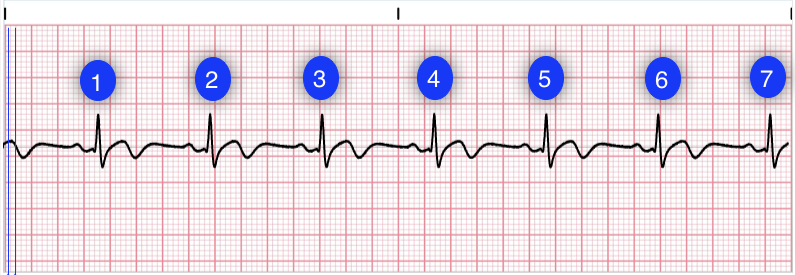# Heart Rate - EKG Basic.

There are several methods for determining heart rate. Our first method is simple. Count the number of QRS complexes over a 6 second interval. Multiply by 10 to determine heart rate. This method works well for both regular and irregular rhythms. In the first image, we can count 7 QRS complexes, so the heart rate is 70.

The second method uses small boxes. Count the number of small boxes for a typical R-R interval. Divide this number into 1500 to determine heart rate. In the second image, the number of small boxes for the R-R interval is 21.5. The heart rate is 1500/21.5, which is 69.8.An error has occurred. This application may no longer respond until reloaded. Reload 🗙